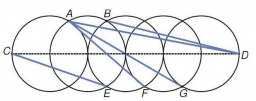# Coordinates 36751

Calculate the circle's length and determine the coordinates of the center of the circle when given its diameter XY, X / -3.2 / and Y / -3, -4 /.

x =  -3
y =  -1
o =  18.8496

### Step-by-step explanation:Did you find an error or inaccuracy? Feel free to write us. Thank you!

Tips for related online calculators
Line slope calculator is helpful for basic calculations in analytic geometry. The coordinates of two points in the plane calculate slope, normal and parametric line equation(s), slope, directional angle, direction vector, the length of the segment, intersections of the coordinate axes, etc.

#### Grade of the word problem:

We encourage you to watch this tutorial video on this math problem: